Download Presentation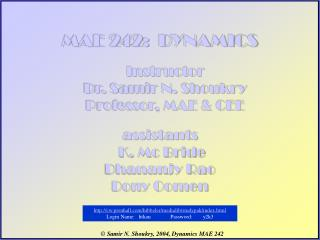MAE 242: DYNAMICS

# MAE 242: DYNAMICS - PowerPoint PPT Presentation

MAE 242: DYNAMICS. Instructor Dr. Samir N. Shoukry Professor, MAE & CEE. assistants K. Mc Bride Dhananjy Rao Dony Oomen. http://cw.prenhall.com/hibbeler/medialib/studypak/index.html Login Name: htkau Password: y2k3. Key to a grade of A in MAE242.I am the owner, or an agent authorized to act on behalf of the owner, of the copyrighted work described.
Download Presentation## MAE 242: DYNAMICS

An Image/Link below is provided (as is) to download presentation

Download Policy: Content on the Website is provided to you AS IS for your information and personal use and may not be sold / licensed / shared on other websites without getting consent from its author.While downloading, if for some reason you are not able to download a presentation, the publisher may have deleted the file from their server.

- - - - - - - - - - - - - - - - - - - - - - - - - - E N D - - - - - - - - - - - - - - - - - - - - - - - - - -
Presentation TranscriptMAE 242: DYNAMICS

Instructor Dr. Samir N. ShoukryProfessor, MAE & CEE

assistants K. Mc BrideDhananjy RaoDony Oomen

http://cw.prenhall.com/hibbeler/medialib/studypak/index.htmlKey to a grade of A in MAE242
• Attend all classes and pay full attention in each class and ask questions. BEFORE YOU COME TO CLASS YOU MUST READ THE RELATED MATERIAL.
• Solve all the book example problems following each class. YOU MUST do using pencil and paper; JUST REDING IS A WASTE OF TIME.
• SOLVE ALL HOMEWORK PROBLEMS: IF YOU CANNOT SOLVE A PROBLEM GO AND ASK QUESTIONS UNTILL YOU SOLVE THE PROBLEM ON YOUR OWN.
• KEEP TIDY RECORD OF THE SOLUTIONS.
• ALWAYS VISIT ESB115 and Ask me, William or McBride.
• REMEMBER: NO ONE WILL EXPLAIN THIS MATERIAL BETTER THAN DR. Shoukry , Dr. William, or Mr. McBride
• YOU HAVE A GREAT OPPORTUNITY …. USE IT.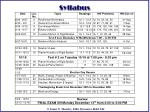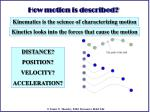How motion is described?

Kinematics is the science of characterizing motion

Kinetics looks into the forces that cause the motion

DISTANCE?

POSITION?

VELOCITY?

ACCELERATION?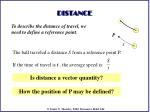P

If the time of travel is t , the average speed is:

DISTANCE

To describe the distance of travel, we need to define a reference point.

The ball traveled a distance S from a reference point P.

Is distance a vector quantity?

How the position of P may be defined?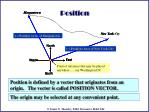Position

Position is defined by a vector that originates from an origin. The vector is called POSITION VECTOR.

The origin may be selected at any convenient point.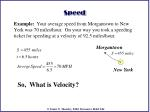Speed

Example: Your average speed from Morgantown to New York was 70 miles/hour. On your way you took a speeding ticket for speeding at a velocity of 92.5 miles/hour.

Morgantown

New York

So, What is Velocity?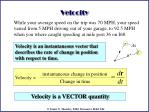instantaneous change in position

Velocity =

dr

Change in time

=

dt

Velocity

While your average speed on the trip was 70 MPH, your speed varied from 5 MPH driving out of your garage, to 92.5 MPH when you where caught speeding at mile post 36 on I68.

Velocity is an instantaneous vector that describes the rate of change in position with respect to time.

Δs

r

r’

Velocity is a VECTOR quantity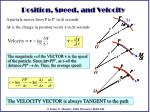r

d

v

=

t

d

r

D

Velocity = v

=

lim

t

D

0

t

O

O

O

Position, Speed, and Velocity

A particle moves from P to P’ in Δt seconds

Δr is the change in position vector r in Δt seconds

The magnitude v of the VECTOR v is the speed of the particle. Since Δr=PP’, as t0 the distance PP’  Δs. Thus, the average speed:

The VELOCITY VECTOR is always TANGENT to the path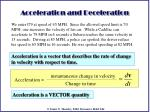instantaneous change in velocity

dv

Acceleration =

=

Change in time

dt

Acceleration and Deceleration

We enter I79 at speed of 45 MPH. Since the allowed speed limit is 70 MPH; one increases the velocity of his car. While a Cadillac can accelerate to 79 MPH in 6 seconds a Subaru reaches the same velocity in 15 seconds. Driving at 85 MPH, a police car spotted; the driver reduce his speed to 65 MPH in 16 seconds. He was spotted speeding at 82 MPH.

Acceleration is a vector that describes the rate of change in velocity with respect to time.

Acceleration is a VECTOR quantitydr

=

=

dt

dt

v

dr

dv

=

=

=

=

v

dt

dt

dr

dr

v

a

dv

dr

=

=

dt

dt

=

=

v

r

a

dt

dv

=

=

a

dv

v

a

dt

dt

=

dr

dv

=

=

r

a

dt

Position, Velocity, & Accelerationdv



=

Integrate

=

Separate variables

f(t) =

f(t)

dt

dv

v

+ C

f(t)

dt

dt





=

+ C

)

r

(

f(t)

dt

dt

+ C’

dv

v2



Integrate

=

Separate variables

=

v

f(r) =

+ C

f(r)

dr

vdv

f(r)

dr

dr

2

dr



dr

1

=

v=f(r) =

dr

t=

dt

+ C’

dt

f(r)

f(r)

Inverse Problem

1. Acceleration is a time function i.e: a=f(t)

2. Acceleration is a time position i.e: a=f(r)C

-1.5

0.5

2.5

0

A

B-25 ft

7 ft

-18 ft

Total distance=7+(7-(-25))-(-25-(-18))=46 ft